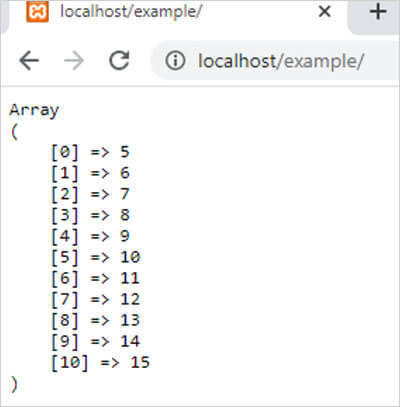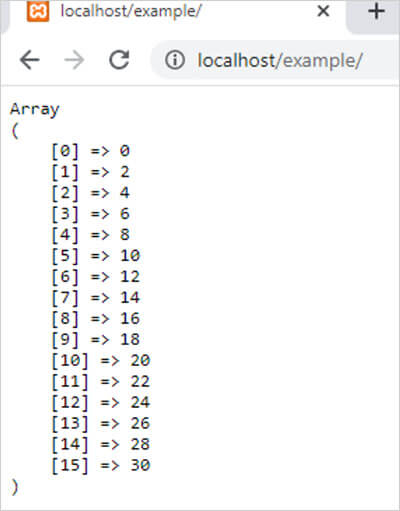range() function in PHP || PHP Range Function

# range() function in php

0 1174

PHP range() function is used to create an array that contains a particular range of items defined by the user. It is an inbuilt function of PHP.

Syntax:

range(\$low, \$high, \$step);

here,

• \$low is the starting point of the range. It is mandatory.
• \$high is the ending point of the array range. It is also mandatory.
• \$step represents the increment value which used to decide the next array element from range. It is optional. By default its value is 1.

Example 2:

```<?php
\$range = range(5,15); // It returns an array which contains values from 5 to 15
echo "<pre>";
print_r (\$range);
?>```

Output:Example 2:

```<?php
\$range = range(0,30,2);  // It returns an array which contains even values from 0 to 30
echo "<pre>";
print_r (\$range);
?>```

Output:Share: